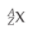×
Get Full Access to Introductory Chemistry - 5 Edition - Chapter 17 - Problem 5q
Get Full Access to Introductory Chemistry - 5 Edition - Chapter 17 - Problem 5q

×

# Explain what each symbol in the notation representsISBN: 9780321910295 34

## Solution for problem 5Q Chapter 17

Introductory Chemistry | 5th Edition

• Textbook Solutions
• 2901 Step-by-step solutions solved by professors and subject experts
• Get 24/7 help from StudySoup virtual teaching assistantsIntroductory Chemistry | 5th Edition

4 5 1 392 Reviews
10
0
Problem 5Q

Explain what each symbol in the notation represents.Step-by-Step Solution:
Step 1 of 3

Solution:

The symbol X represents , symbol of an atom

A represents mass number of an atom

Z represents the atomic number.

For example

1123Na , where X= Na, A = 23 and Z = 11

Step 2 of 3

Step 3 of 3

##### ISBN: 9780321910295

This textbook survival guide was created for the textbook: Introductory Chemistry, edition: 5. This full solution covers the following key subjects: explain, notation, represents, symbol. This expansive textbook survival guide covers 19 chapters, and 2046 solutions. The full step-by-step solution to problem: 5Q from chapter: 17 was answered by , our top Chemistry solution expert on 05/06/17, 06:45PM. Since the solution to 5Q from 17 chapter was answered, more than 376 students have viewed the full step-by-step answer. The answer to “?Explain what each symbol in the notation represents.” is broken down into a number of easy to follow steps, and 8 words. Introductory Chemistry was written by and is associated to the ISBN: 9780321910295.

Unlock Textbook Solution